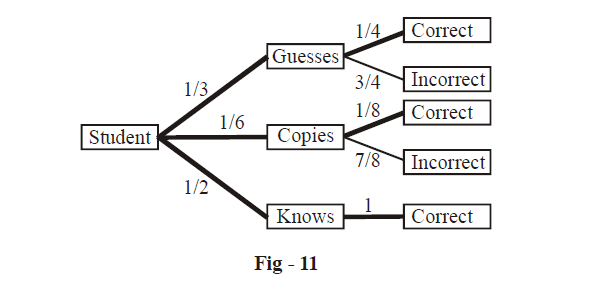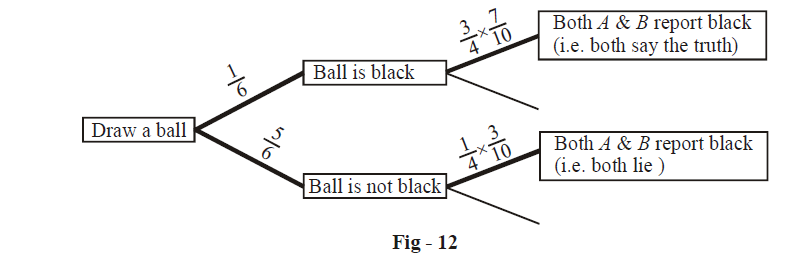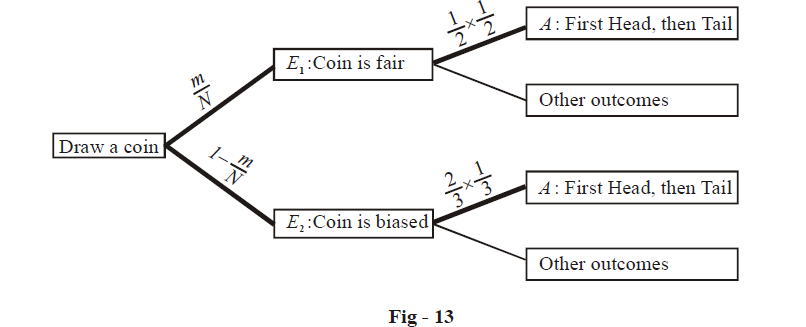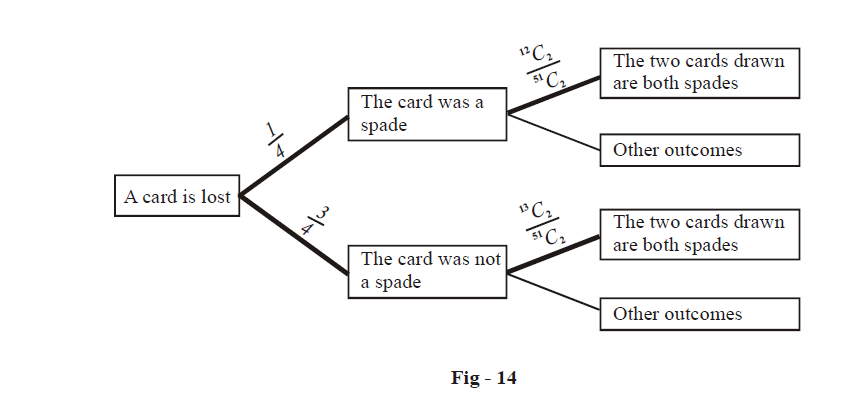# Examples on Inverse Probability and Bayes Theorem

Go back to  'Probability'

Example – 13

In an objective type examination, each question has four options of which only one is correct. A student taking the examination either guesses or copies or knows the answer to any question. The probability that he guesses is \begin{align}\frac{1}{3}\end{align} and that the he copies is \begin{align}\frac{1}{6}\end{align} . The probability that he answers correctly if he copies is  \begin{align}\frac{1}{8}\end{align}. If an answer is given to be correct, find the probability that the student knew the answer.

Solution: We see that the student can make three moves, with associated probabilities as:

$P\left( \text{makes a guess} \right)=\frac{1}{3},P\left( \text{copies} \right)=\frac{1}{6},P\left( \text{knows} \right)=1-\left( \frac{1}{3}+\frac{1}{6} \right)=\frac{1}{2}$

Now, if he copies, we know that the probability of his answer being correct is $$\frac{1}{8}$$. Also, if he guesses, he’ll select any of the four options with equal likelihood, which means that the probability of his answer being correct is $$\frac{1}{4}$$ .

Thus, we have the following probability tree:We see that we have three paths leading to the correct answer, and we want to find the probability of the bottomost path, that is the probability that the student knew the answer given that he answered it correctly. This probability is now simply:

\begin{align}& P\left( \text{knew / answered correctly} \right)=\frac{\frac{1}{2}\times 1}{\frac{1}{3}\times \frac{1}{4}+\frac{1}{6}\times \frac{1}{8}+\frac{1}{2}\times 1} \\ &\qquad\qquad\qquad\qquad\qquad\qquad\quad =\frac{24}{29} \\ \end{align}

Can you appreciate why this value is so high (greater than 80%)?

Example – 14

A speaks truth 3 times out of 4 while B does so 7 times out of 10. A ball is drawn at random from a bag containing one black ball and five other balls of different colors. Both A and B report that a black ball has been drawn from the bag. Find the probability of their assertion being true.

Solution: To slightly rephrase the problem, we’ve to find the probability of the ball actually being black given that both A and B report it to be black.

The probability tree can easily be drawn (partially shown here, only the paths relevant to us have been completed):We want to find the probability of the upper path which is:

\begin{align}& P\left\{ \left. \left( \text{Ball is black} \right)\,\, \right|\,\left( \text{Both}\,A\;\And B\;\text{report it to be black} \right) \right\}=\frac{\frac{1}{6}\times \left( \frac{3}{4}\times \frac{7}{10} \right)}{\frac{1}{6}\times \left( \frac{3}{4}\times \frac{7}{10} \right)+\frac{5}{6}\times \left( \frac{1}{4}\times \frac{3}{10} \right)} \\ &\qquad\qquad\qquad\qquad\qquad\qquad\qquad\qquad\qquad\qquad\qquad\;\;\;\;=\frac{7}{12} \\ \end{align}

Let us now rewrite the solution in standard terminology. Let us define the following events:

B : Black ball is drawn from the bag

X : Both A and B report black.

Thus, observe that we need to find P(B/X) which is by the Baye’s theorem (in fact compare this relation with the tree diagram in Fig-12 and see how the two correspond)

\begin{align} & P\left( B/X \right)=\frac{P\left( B \right)P\left( X/B \right)}{P\left( X \right)} \\ &\qquad\quad\;\;=\frac{P\left( B \right)P\left( X/B \right)}{P\left( B \right)P\left( X/B \right)+P\left( {\bar{B}} \right)P\left( X/\bar{B} \right)} \\ & \qquad\quad\;\;=\frac{\frac{1}{6}\times \left( \frac{3}{4}\times \frac{7}{10} \right)}{\frac{1}{6}\times \left( \frac{3}{4}\times \frac{7}{10} \right)+\frac{5}{6}\times \left( \frac{1}{4}\times \frac{3}{10} \right)} \\ & \qquad\quad\;\;=\frac{7}{12} \\ \end{align}

Example – 15

A box contains N coins, m of which are fair and the rest are biased. The probability of getting Head when a biased coin is tossed is \begin{align}\frac{2}{3}\end{align}. A coin is drawn at random from the box and tossed twice. The first time it shows Head and the second time it shows Tail. What is the probability that the coin drawn is fair?

Solution: The probability tree for this problem is as follows:The required probability p is

\begin{align}& p=\frac{\frac{m}{N}\times \left( \frac{1}{2}\times \frac{1}{2} \right)}{\frac{m}{N}\times \left( \frac{1}{2}\times \frac{1}{2} \right)+\left( 1-\frac{m}{N} \right)\times \left( \frac{2}{3}\times \frac{1}{3} \right)} \\ &\; =\frac{\frac{m}{4\,N}}{\frac{m}{4\,N}+\frac{2N-2m}{9N}} \\ &\; =\frac{9\,m}{m+8\,N} \\ \end{align}

In the terminology of Baye’s theorem, the expression for p will be

$p=P\left( {{E}_{1}}/A \right)=\frac{P\left( {{E}_{1}} \right)\cdot P\left( A/{{E}_{1}} \right)}{P\left( {{E}_{1}} \right)\cdot P\left( A/{{E}_{1}} \right)+P\left( {{E}_{2}} \right)\cdot P\left( A/{{E}_{2}} \right)}$

Example – 16

A card from a pack of 52 cards is lost. From the remaining cards of the pack two cards are drawn at random and are found to be spades. Find the probability that the missing card was a spade.

Solution: Again, let us first approach the problem by drawing the corresponding probability tree:Observe carefully the probabilities of all the relevant steps.

The required probability p is

\begin{align} & p=\frac{\frac{1}{4}\times \frac{^{\text{12}}{{C}_{\text{2}}}}{^{\text{51}}{{C}_{\text{2}}}}}{\frac{\text{1}}{\text{4}}\times \frac{^{\text{12}}{{C}_{\text{2}}}}{^{\text{51}}{{C}_{\text{2}}}}+\frac{3}{4}\times \frac{^{\text{13}}{{C}_{\text{2}}}}{^{\text{51}}{{C}_{\text{2}}}}} \\ &\;\; =\frac{11}{50} \\ \end{align}

Hence, the chances of the missing card being a spade are 22%. Can you appreciate this answer? In particular, can you see why it is less than 25%?

## TRY YOURSELF - III

Q. 1 A bag contains two balls each of which is either white or black. A white ball is added to the bag. What is the probability of drawing a white ball from the bag now?

Q. 2 Three factories A, B, C produce the same product. The factory A produces twice as many products as B and C. It is known that 2% of the products of A as well as C are defective while 4% of the products of B are defective. If all the products from the three factories are stocked together and one is drawn at random, what is the probability that it is defective?

Q. 3 A man has three coins A, B and C. A is fair, while B and C have probabilities \begin{align}\frac{2}{3}\,\,\text{and}\,\,\frac{1}{3}\end{align} respectively of showing Heads. A coin is chosen at random and tossed three times giving two Heads and one Tail, in that sequence. Find the probability that A was chosen.

Q. 4 The probabilities of A, B and C hitting a target upon firing once are \begin{align}\frac{4}{5}\,,\,\frac{3}{4}\,\,\text{and}\,\,\frac{2}{3}\end{align} respectively. They fire once each and two of them hit while one misses the target. What is the probability that C was the one who missed?

Q. 5 In a bag there are six balls of unknown colors; three balls are drawn at random and found to be all black. Find the probability that no black ball is left in the bag. In other words, you have to find the probability that the bag contained exactly three black balls.

Probability
grade 11 | Questions Set 1
Probability
Probability
grade 11 | Questions Set 2
Probability